# Hooke's Law and Simple Harmonic Motion

## Objective

• to measure the spring constant of the springs using Hooke's Law
• to explore the static properties of springy objects and springs, connected in series and parallel
• to study the simple harmonic oscillator constructed from springs and masses
• to verify that the period of the SHM is proportional to the square root of the mass and independent of the amplitude
• to measure the dynamic spring constant
• to verify the conservation of energy law

## Equipment

### Part I:

• two nearly identical springs
• long rubber band
• support stand with a meter stick
• 50 g mass hanger
• set of masses from 100 g up to 600 g
• balance scale
• Science Workshop Interface with force sensor and rotational motion detector used as a linear sensor

### Part II:

• collision cart of a known mass on a horizontal dynamics track oscillating by the means of springs in parallel
• motion sensor and photogate connected to the Science Workshop interface
• non-linear springy objects (rubber bands)
• two rectangular weights of ~0.5 kg each to change the mass of the system

## Introduction and Theory

#### Hooke's Law

Elastic force occurs in the spring when the spring is being stretched/compressed or deformed (Δx) by the external force. Elastic force acts in the opposite direction of the external force. It tries to bring the deformed end of the spring to the original (equilibrium) position. See fig. 1.Figure 1

If the stretch is relatively small, the magnitude of the elastic force is directly proportionally to the stretch Δx according to Hooke's Law:
( 1 )
Fel = −kΔx
where k is a constant, usually called spring constant, and Δx is a stretch (the difference between new (x) and equilibrium position). The minus sign in front of the spring constant in equation 1 indicates that the elastic force and stretch act in the opposite direction.

#### Simple Harmonic Motion

If the hanging mass is displaced from the equilibrium position and released, then simple harmonic motion (SHM) will occur. SHM means that position changes with a sinusoidal dependence on time.
( 2 )
x = Xmax cos(ωt
The following are the equations for velocity and acceleration.
( 3 )
v = −Xmaxω sin(ωt
( 4 )
a = −Xmaxω2 cos(ωt
By substituting equations 2, 4 and 1 into Newton's Second Law, one can derive the equation for the angular resonant frequency of the oscillating system:
( 5 )
ω =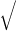k m

where k is the spring constant and m the mass of the system undergoing the simple harmonic motion. The unit of angular frequency is
The natural resonant frequency of the oscillator can be changed by changing either the spring constant or the oscillating mass. Using a stiffer spring would increase the frequency of the oscillating system. Adding mass to the system would decrease its resonant frequency. Two other important characteristics of the oscillation system are period (T) and linear frequency (f). The period of the oscillations is the time it takes an object to complete one oscillation. Linear frequency is the number of the oscillations per one second. The period is inversely proportional to the linear frequency.
( 6 )
T =
 1 f

The unit of the period is a second (s) and the unit of the frequency is Hertz or s–1 (Hz = 1/s). The angular frequency is related to the period and linear frequency according to the following expression.
( 7 )
ω = 2πf =
 2π T

#### Energy

In order for the oscillation to occur, the energy has to be transferred into the system. When an object gets displaced out of equilibrium, then elastic potential energy is being stored in the system. After the object is released, the potential energy transforms into kinetic energy and back. In the harmonic oscillator, there is a continuous swapping back and forth between potential and kinetic energy. For an oscillating spring, its potential energy (Ep) at any instant of time equals the work (W) done in stretching the spring to a corresponding displacement x.
( 8 )
Ep = W =
 1 2
kx2
The kinetic energy (Ek) of the oscillator for any instance of time will follow the well-known equation:
( 9 )
Ek =
 1 2
mv2
The maximum potential energy and maximum kinetic energy can be calculated accordingly.
( 10 )
Ep =
 1 2
kXmax2
( 11 )
Ek =
 1 2
kVmax2
According to the law of conservation of energy: "The mechanical energy is conserved (neither destroyed nor created) in the frictionless oscillating system."

## Procedure

Please print the worksheet for this lab. You will need this sheet to record your data.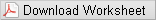### Part I - Hooke's Law

#### Measurement of a spring constant, method 1

The purpose of this part of the laboratory activity is to find the spring constant of the spring. The spring constant is a coefficient of proportionality between elastic force and displacement, according to Hooke's Law (equation 1).
1
Hang a spring from the support, add a weight hanger, and measure the initial equilibrium position with the meter stick and record it.
2
Add masses in steps of 100 g up to 600 g and measure the corresponding position.
3
Discuss with your group members the columns that need to be prepared in GA to record the data. Make a draft of a table and check it with your TA. Prepare the columns in GA.
4
Create a new calculated column for the elastic force data (DATA → NEW CALCULATED COLUMN → equation: F = variables "m"*g, where m is in kg).
5
Create a new calculated column for displacement (DATA → NEW CALCULATED COLUMN → equation = variables "position" — initial equilibrium position).
6
Make a plot of force vs. displacement.
7
Record the slope of the graph and its uncertainty in the Lab 8 Worksheet. You will use the value of slope and its uncertainty to find the spring constant and its error.

#### Measurement of spring constant, method 2

The apparatus setup shown in fig. 2 will be used to find the spring constant in spring 2.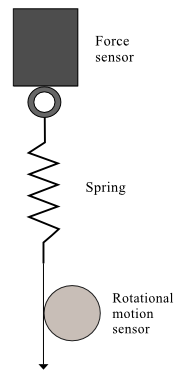Figure 2: The apparatus setup for the Hooke's Law experiment

1
Open the pre-set experiment file: desktop\pirt-labs\phy 113\PreSetUpFiles\Springs.
2
Before taking the actual data, calibrate the force sensor. Click the "Setup" button in the toolbar. Then click on the force sensor icon and press the "Calibrate Sensors" button. This brings up the calibration panel shown in fig. 3.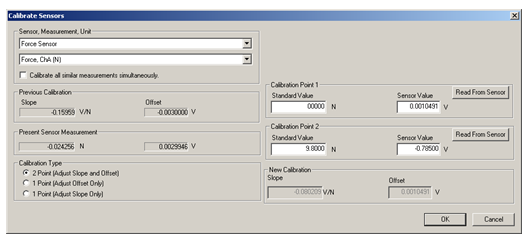Figure 3: Calibration of force sensor in Data Studio

The "2 Point" option should be selected as the "Calibration Type". With no load on the force sensor, enter 0 in the "Calibration Point 1" standard value window. Push the "Tare" button on the force sensor. This action adjusts the force sensor reading to zero. Press "Read From Sensor" button. Next hang the 1 kg hooked mass on the sensor and type 9.8 in the "Calibration Point 2" standard value window. Click on the "Read From Sensor" button. Click on "OK" to save this calibration. Close the "Calibrate Sensors" and the "Setup" windows. Now you are ready to take the actual measurements of the spring constants.
3
Replace the 1 kg weight with the spring. Attach a piece of string to it. Wrap the string around the large pulley of the rotational motion detector in a counter clockwise direction as depicted in fig. 2. The rotational motion sensor has been calibrated to read the linear distance.
4
Press the "Start" button to initiate the data acquisition. Carefully pull on the string, watching the Force display window. Start releasing the string when the force reaches up to 10 N.
5
Apply the linear fit to the good part of your recording (see fig. 4) which presents elastic force vs displacement graph. The slope of this line gives the spring constant.
6
Record the slope of the Force vs potion graph and its uncertainty in the Lab 8 Worksheet. You will use the value of slope and its uncertainty to find the spring constant and its error.
7
Repeat this procedure for a system of springs in series and in parallel.
8
Record the slope of the Force vs position graph and its uncertainty in the Lab 8 Worksheet. You will use the value of slope and its uncertainty to find the spring constant and its error. In the Discussion section, you will need to compare the value of the spring constants for the system of springs to the value of each spring constant.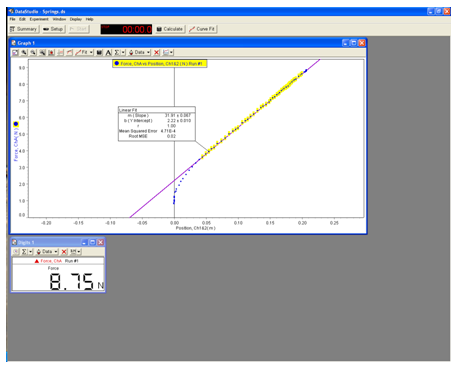Figure 4: A sample of experiment file in DataStudio

#### Investigation of nonlinear springy object.

1
In the experimental setup from fig. 2, replace the spring with the long rubber band.
2
Record force vs. position for both down (increasing force) and up (decreasing force) strokes.
3
Use this data to address whether or not the rubber band follows Hooke's Law (equation 1).

### Part II - Simple Harmonic Motion

In this part of the experiment you will verify if the period depends on the amplitude; calculate the resonance frequency and spring constant of a system. You will record the collected data in the Lab 8 Worksheet.
1
Setup the experiment as shown on the pictures below. Open the pre-set experiment file: desktop\pirt-labs\phy 113\PreSetUpFiles\SHM.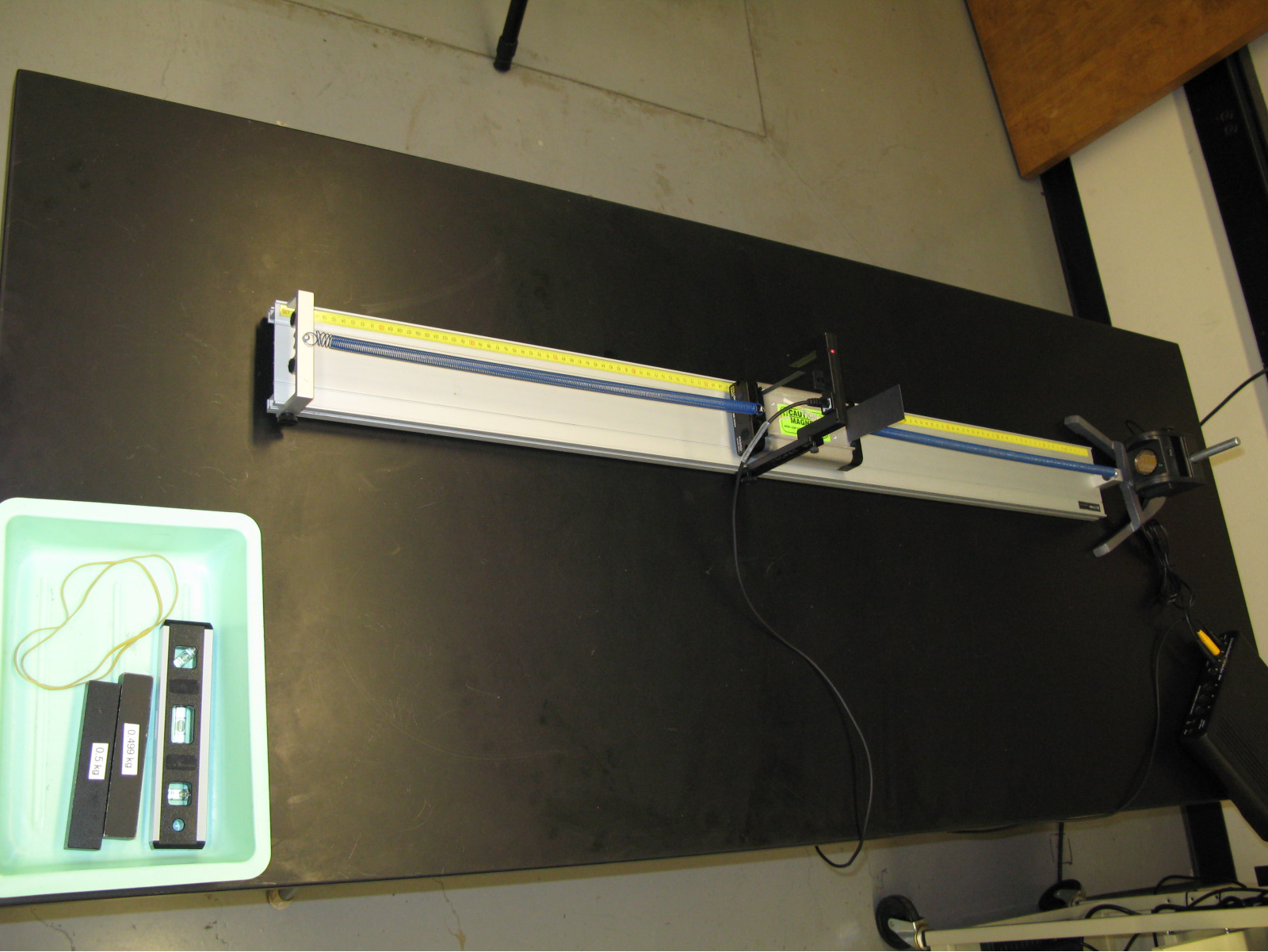Figure 5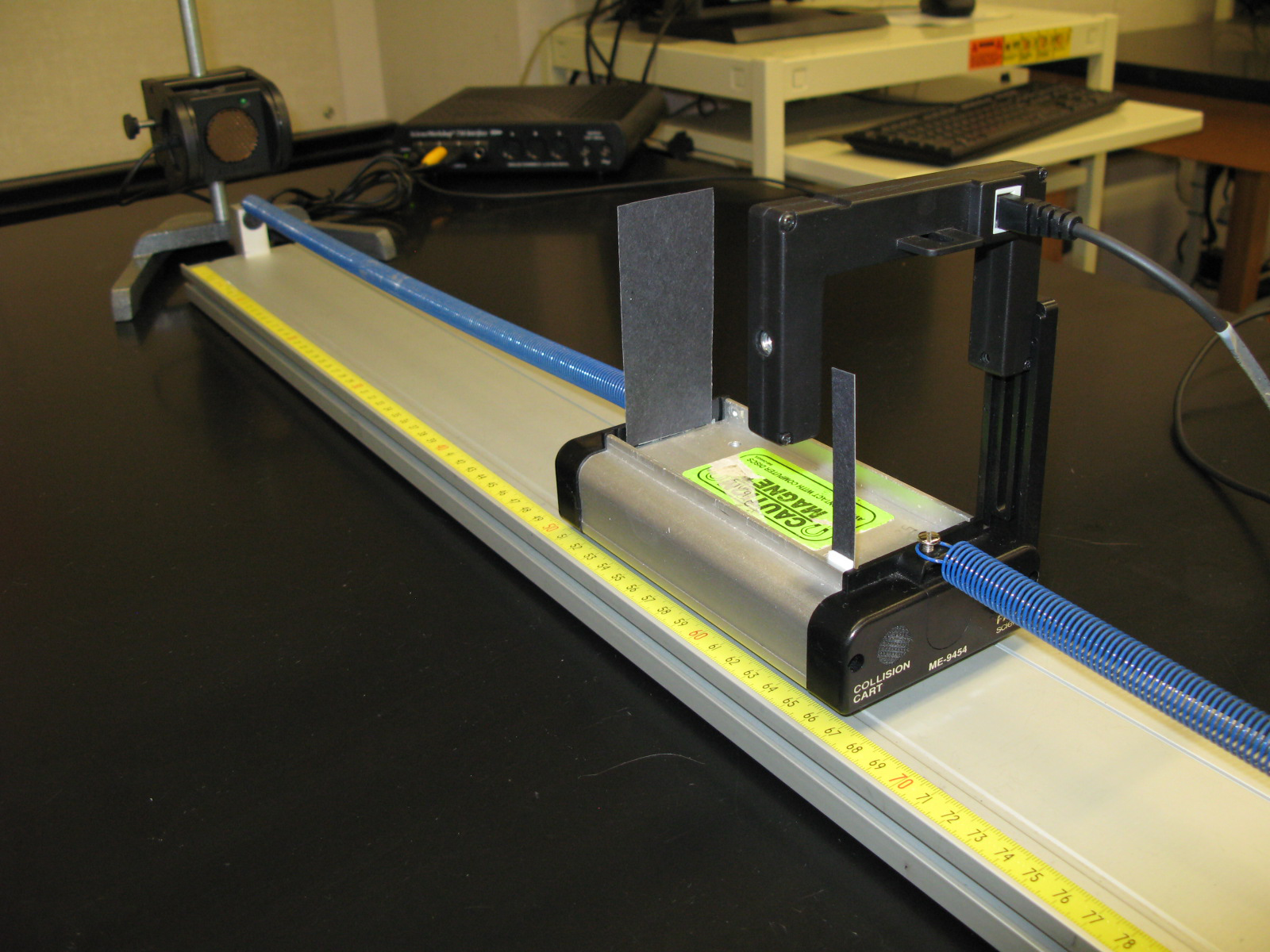Figure 6

2
With no added mass, measure the period of oscillations for starting amplitudes of 4 cm and 12 cm. Data acquisition will stop automatically after 5 sec. The sample recording is shown in fig. 2. The period is measured by the photogate and recorded in the table 1 to the left of the graph.
3
With no added mass, displace the cart from its equilibrium position about 8 cm and start the recording. Record again the period of oscillations of a cart measured by the photogate. Record all three periods of oscillations in the Lab 8 Worksheet.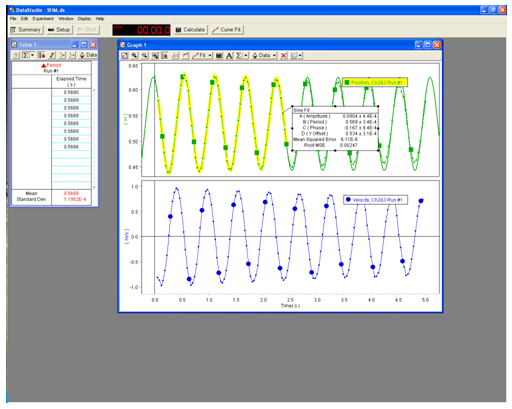Figure 7: A sample file for SHM experiment in DataStudio

4
Fit the recording of position vs. time to the sine wave. Parameter A is amplitude (maximum displacement) of the oscillations. Parameter B gives you the period of oscillations. You can compare its value to the value of the period measured by photogate. Parameter D — is the equilibrium position. Use parameter B to calculate resonance frequency and spring constant of a system using equations 7 and 5 from the Introduction and Theory in this Lab Manual.
5
Fit the recording of velocity vs. time to the sine wave. Record the parameter A — the amplitude (maximum value) of the velocity vs time graph.
Notice that both graphs, position vs. time and velocity vs. time, are periodic waves of the same frequency just shifted by 90° or
 π 2
The maximum and minimum values of the position occur when the velocity is zero, and likewise the maximum and minimum values of velocity happen when the position is at its equilibrium.
In terms of the energy of the system, it means that when the potential energy is a maximum (maximum displacement) the kinetic energy is zero. At this moment, energy is all potential. Likewise at the point when the spring is not stretched (the object passes an equilibrium point), the energy is all kinetic. To verify the conservation of energy theorem for the oscillating cart, it is sufficient to compare its maximum potential energy with its maximum kinetic energy by finding the percent difference of the energy loss.
( 12 )
%loss =
 PEi − KEf PEi
· 100%

#### Relationship between period and mass

This is the part of the experiment where you will verify that the period of the SHM is proportional to the square root of the mass.
1
Load the cart with heavy masses (one at a time) and measure the period of SHM while keeping the amplitude constant, e.g. 8 cm.
2
Including the data from the previous part of the experiment, you will have three points to make a graph of period vs.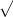m
.
According to the theory (equations 5 and 7) this graph should be a straight line.
3
Record the slope of the T vsm

graph in the Lab 8 Worksheet.
4
Use the value of slope and its uncertainty to find the spring constant.
5
Based on the slope of the graph calculate the dynamic spring constant of the system.
Hint: Substituting
• ω =  2π T

into
• ω =k m

gives
•  2π T
=k m
.

Solving for T yields the following.
• T =
2πkm

## Discussion

State the purpose of the lab. Compare the experimental results of a spring constant for a single spring from methods 1 and 2 to their theoretical values. What is the percent discrepancy? How is the spring constant for a system of two springs in series related to the individual spring constants? How is the spring constant for a system of two springs in parallel related to the individual spring constants? Do all spring like objects obey Hooke's Law? What conclusion can be made about the relationship between period and amplitude based on the collected data? Are the measurements of the spring constant consistent with each other? Was the Law of Conservation of Energy hold in your experiment? Based on your experimental data, what is the relationship between the period of oscillaions and mass in the spring-mass system? Explain what physical quantity can be taken as a characteristic of the oscillating system. Summarize the main characteristics of the simple harmonic motion.

## Conclusion

Have you met the objective of the lab?